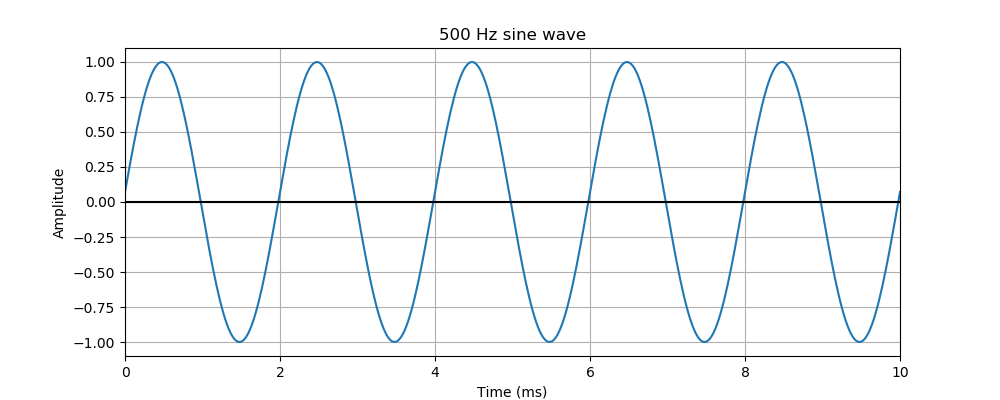# Pysound simple sine waves

Martin McBride
2021-09-30

In this example, we will create a simple sine wave.

## The code

Here is the code:

from pysound.buffer import BufferParams
from pysound.oscillators import sine_wave
from pysound import soundfile
from pysound.graphs import Plotter

params = BufferParams()
out = sine_wave(params, frequency=500)
soundfile.save(params, 'sine-500.wav', out)


After importing several modules, there are just 3 lines of code.

First, we create a BufferParams object. This object controls the length of the sound, and also the sample rate. The default constructor specifies a sound that is 1 second long, and has a sample rate of 44,100 samples per second (this is the sample rate used by an audio CD):

params = BufferParams()


Next, we call the sine_wave function, which creates a sine wave tone.

out = sine_wave(params, frequency=500)


We pass in the params object to tell the function how long the sound should last, and what sample rate to use.

We also pass in a frequency of 500, to set the wave frequency to 500 Hz.

The sine_wave function takes several other parameters too, but we will use the default values. This creates a sine wave tone with an audio frequency of 500 Hz and an amplitude of 1.0.

The function returns a NumPy array, that we store in out.

Finally, we write the data out to a WAV file:

soundfile.save(params, 'sine-500.wav', out)


This takes the data and converts it to WAV format. It is stored as sine-500.wav in the current folder.

## Listening to the sound

You should be able to play the wav file using your system media player.

You can also use Audacity, an open-source audio editor. This is a very useful program that can be used to play, view and edit sound files, it is well worth installing.

## Plotting the wave

The Pysound graphs module allows us to create a plot of a sound buffer.

We use the Plotter object to create a graph of the first part of the wave:

## Plot a graph of the first part of the previous sine wave
Plotter(params, 'sine-500.png', out).with_timerange(0, 0.01)\
.with_title("500 Hz sine wave")\
.in_milliseconds()\
.plot()


The Plotter constructor accepts params and out from the previous code, and also the string 'sine-500.png' which tell Pysound where to store the image file.

We also set the time range, so that the plot only includes the first 0.01 seconds of the wave.

Since the wave has a frequency of 500 Hz, one cycle of the wave takes 0.002 seconds (ie, one 500th of a second). This means that the first 0.01 seconds of the waveform contains 5 full cycles of the wave. Here is the graph:The graph is scaled in milliseconds (ms) as this is easier than dealing with small fractions. 0.01 seconds is 10 ms, 0.002 seconds is 2 ms.

If we tried to plot the whole one second of the wave, we wouldn't be able to see the individual waves, they would be far too close together. By plotting a 10 ms section of the wave, the waveform can be seen easily.

For a full description, see the later tutorial on the graphs module.